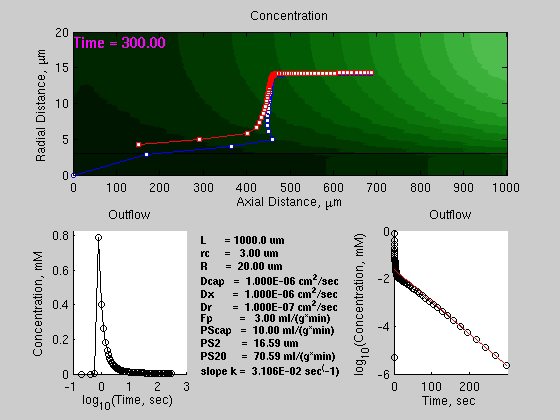Model number
0327

A two region model of capillary and cell with cell subdivided into 20 subregions with axial and radial diffusion. Time-dependent plots of contours and center-of-mass of material are calculated using MATLAB.

## Description

```   This is a modification of the BTEX20 model. Radial diffusion
has been included by subdividing the tissue region into 20
layers and solving the 2-D time dependent problem. The model
includes a java interface to Matlab(TM) to make a movie (avi file)
of the concentration contours in the capillary and tissue as
a function of time. In order to use this feature, the user
must have the Matlab(TM) routines and this JSim project file in
the user's directory, along with the Matlab(TM) path set to that
directory. The two lines of code at the end of this model
which call the java interface to Matlab(TM) have been commented out
(see code above). The Matlab routines are contained in a
the project file.

```

The following graphs are a snapshot at the end of the model run of contours of the concentration in the capillary and surrounding tissue. Top panel shows the center of Mass of the substance (red tissue only, blue tissue+capillary).
Panel Bottom Left shows the outflow on a log(Time)-linear(Concentration) plot. Panel Bottom Right shows the outflow on a linear(Time)-log(Concentration) plot. Parameters for this run are indicated in the middle of the Bottom Panel.

## Equations

The equations for this model may be viewed by running the JSim model applet and clicking on the Source tab at the bottom left of JSim's Run Time graphical user interface. The equations are written in JSim's Mathematical Modeling Language (MML). See the Introduction to MML and the MML Reference Manual. Additional documentation for MML can be found by using the search option at the Physiome home page.Blue-white curve is the center of mass including the capillary.
Red-white curve is the center of mass excluding the capillary.

References

```W.C. Sangren and C.W. Sheppard.  A mathematical derivation of the
exchange of a labelled substance between a liquid flowing in a
vessel and an external compartment.  Bull Math BioPhys, 15, 387-394,
1953.

C.A. Goresky, W.H. Ziegler, and G.G. Bach. Capillary exchange modeling:
Barrier-limited and flow-limited distribution. Circ Res 27: 739-764, 1970.

J.B. Bassingthwaighte. A concurrent flow model for extraction
during transcapillary passage.  Circ Res 35:483-503, 1974.

B. Guller, T. Yipintsoi, A.L. Orvis, and J.B. Bassingthwaighte. Myocardial
sodium extraction at varied coronary flows in the dog: Estimation of
capillary permeability by residue and outflow detection. Circ Res 37: 359-378, 1975.

C.P. Rose, C.A. Goresky, and G.G. Bach.  The capillary and
sarcolemmal barriers in the heart--an exploration of labelled water
permeability.  Circ Res 41: 515, 1977.

J.B. Bassingthwaighte, C.Y. Wang, and I.S. Chan.  Blood-tissue
exchange via transport and transformation by endothelial cells.
Circ. Res. 65:997-1020, 1989.

Poulain CA, Finlayson BA, Bassingthwaighte JB.,Efficient numerical methods
for nonlinear-facilitated transport and exchange in a blood-tissue exchange
unit, Ann Biomed Eng. 1997 May-Jun;25(3):547-64.
```

Modular Program Constructor (MPC)

Key terms
plasma
cell
2-d